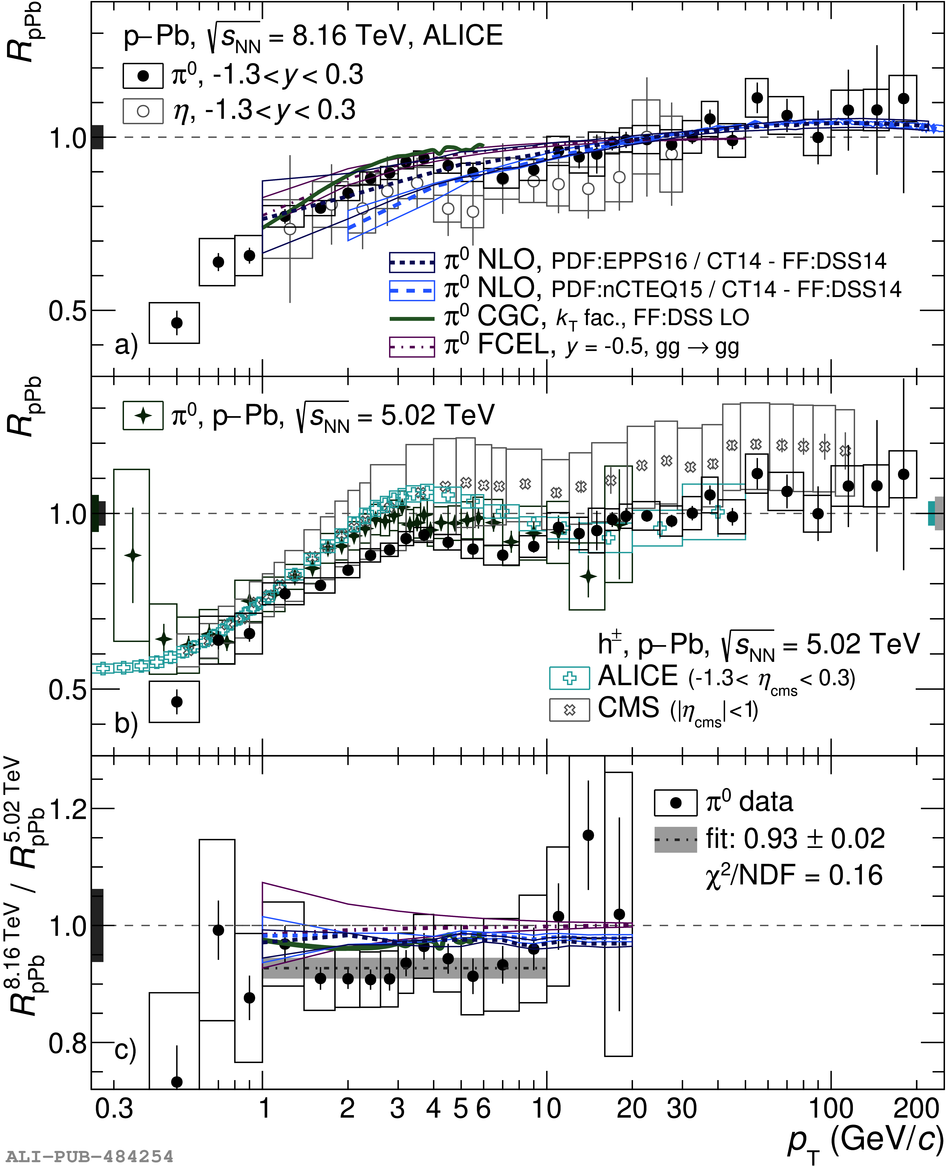# Figure 2

 < p>a)~$\rpp$ for $\pz$ and $\eta$ mesons in \pPb\ collisions at $\sU$ together with NLO~, CGC~ and FCEL~ predictions b)~$\rpp$ for $\pz$ at $\sU$ compared with $\pz$~ and charged hadron measurements~ at $\snn=5.02$ TeV c)~Ratio of the $\pz$ $\rpp$ at $\sU$ to that at $\snn=5.02$ TeV together with corresponding CGC and FCEL model predictions Statistical uncertainties are shown as vertical bars; the systematic uncertainties as boxes. The overall normalization uncertainties are indicated as solid boxes around unity and amount to 3.4\% in a) and b), and to 6.2\% in c).< /p>